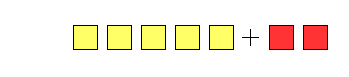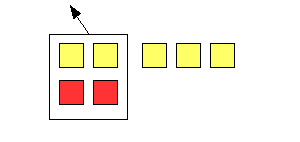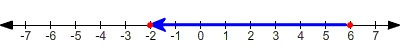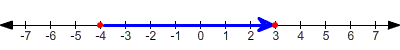# Adding and Subtracting with Negatives

Adding any number to its opposite —also called the additive inverse—always gives zero as the result. For example:

$\begin{array}{l}-999+999=0\\ 2.5+\left(-2.5\right)=0\\ 1+\left(-1\right)=0\end{array}$

Once you know this, there are several ways to think about addition.

### Algebra Tile Method

Let yellow tiles represent positive numbers, and red tiles represent negative numbers.

Example 1:

The addition problem $5+\left(-2\right)$ can be represented asGroup the two negative tiles with two positive tiles.Since $2+\left(-2\right)=0$ , these tiles disappear. We are left with $3$ positive tiles.So $5+\left(-2\right)=3$ .

When both numbers are negative , we have only negative tiles, so the answer is also negative.

Example 2:

The addition problem $-3+\left(-4\right)$ can be represented asThe result is simply $7$ negatives tiles.So $-3+\left(-4\right)=-7$ .

### Number Line Method

When you add a positive number, you move to the right on the number line.

When you add a negative number, you move to the left on the number line.

Example 3:

Add $6+\left(-8\right)$ using a number line.

Start at $6$ , and move $8$ units to the left.$6+\left(-8\right)=-2$

Subtracting a number is the same as adding its opposite.

So, subtracting a positive number is like adding a negative; you move to the left on the number line.

Subtracting a negative number is like adding a positive; you move to the right on the number line.

Example 4:

Subtract $-4-\left(-7\right)$ .

Start at $-4$ , and move $7$ units to the right.$-4-\left(-7\right)=3$ .